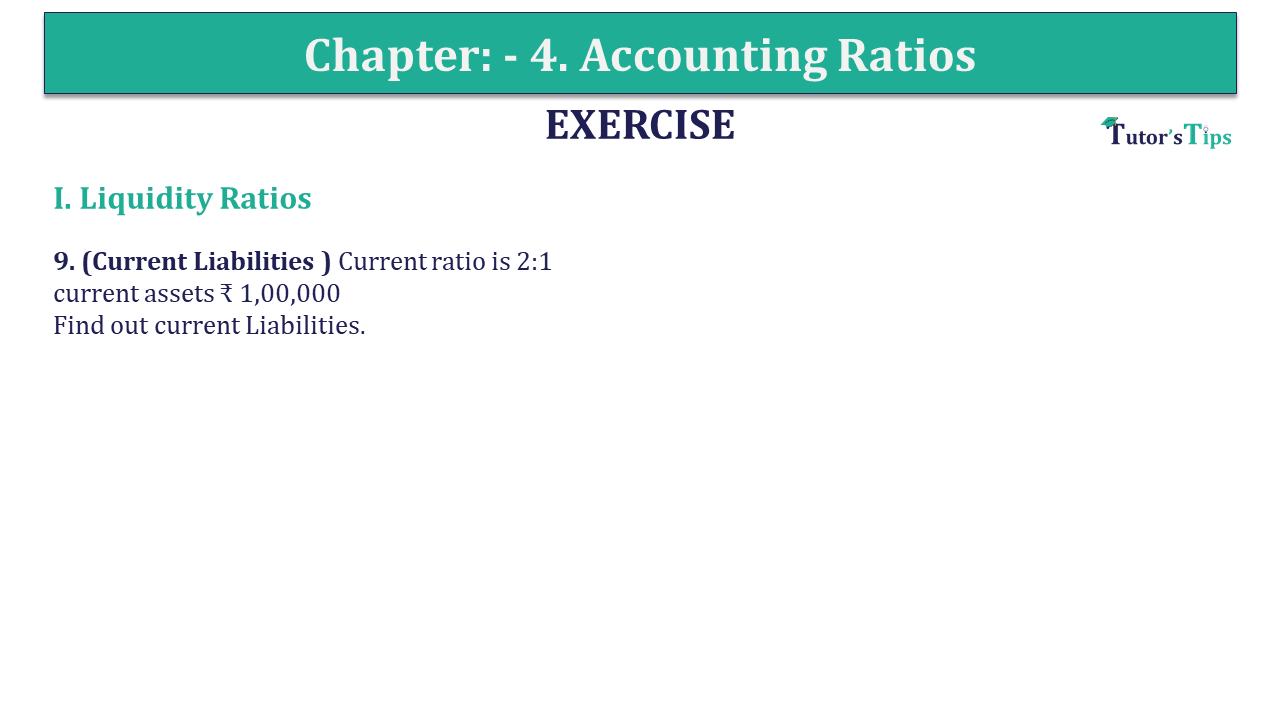# Question 09 Chapter 4 of +2-B – USHA Publication 12 ClassQ-9- CH-4 Book 2 - Usha Pub. +2 Book 2020 - Solution

Question 09 Chapter 4 of +2-B

I. Liquidity Ratios

9. (Current Liabilities ) Current ratio is 2:1
current assets ₹ 1,00,000
Find out current Liabilities.

## The solution of Question 09 Chapter 4 of +2-B: –

 Current Assets = ₹ 1,00,000 Current Ratio = 2: 1

 Current Ratio= Current Assets Current Liabilities

 2 = ₹ 1,00,000 Current Liabilities
 Current Liabilities = ₹ 1,00,000 2 = ₹ 50,000

What are Liquidity Ratios – Formulas and Examples

Comment if you have any question.

Also, Check out the solved question of previous Chapters: –